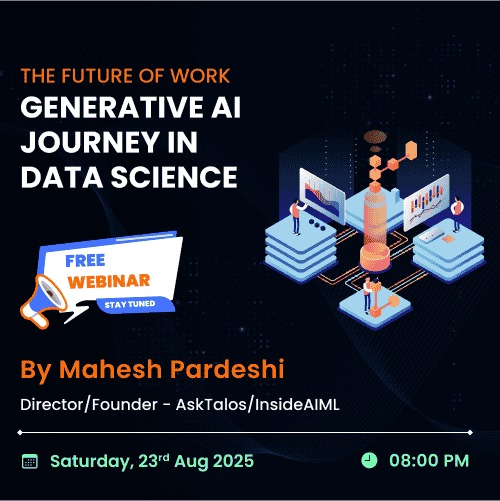All Courses#### Generative AI Journey in Data ScienceDec 6th (8:00 PM) 1972 Registered
More webinars

# Introduction to Machine LearningAshish Katri

2 years ago• What’s is Machine Learning?
• Machine Learning -
1. Supervised Learning.
2. Unsupervised Learning.
3. Reinforcement Learning

Let’s first understand an overview of Machine Learning then we learn more in details about Linear Regression.

## What’s is Machine Learning?

Here, I will not give the theoretical definition. I will try give you a simple definition of Machine Learning.
Machine Learning: It is the field of Computer Science that gives computer’s the ability to learn without being explicitly programmed.
Now I know you may have a doubt that then what’s the difference between Traditional programming Vs Machine Learning.In Traditional programming, we give data as an input and also the program/tasks to be done to the computer and then the computer follows the program/instructions and produces the outputs.

## Machine Learning

In Machine learning, we provide data and its output as an input to the computer and try to find a program (trained model) which can be used to predict the output of the other data points.
Machine Learning is broadly divided into 3 types:

### 1. Supervised Learning

It is a task of learning a function/equation that maps an input to output based on the given set of “input-output” pair.
Further, Supervised Learning is classified into 2 types:
• Regression: It’s a technique to find a best-fitting line to the given data points.
• Classification: It’s a technique to a linear or non-linear Separator to the given data points.

### 2. Unsupervised Learning

It is a study of how systems can infer a function to describe a hidden structure/pattern from the unlabeled data. Here we will only have input data and no corresponding output data.
Unsupervised learning is further divided into 2 types:
• Clustering: It’s a process to discover hidden groupings in the data points.
• Association: It’s a process to discover the rules that describe the huge data.

### 3. Reinforcement Learning

A reinforcement learning algorithm, or agent, learns by interacting with its environment. The agent receives rewards by performing correctly and penalties for performing incorrectly. The agent learns without intervention from a human by maximizing its reward and minimizing its penalty.
This is a very small introduction to Machine Learning and its types. In further articles and tutorials, you will find in-depth knowledge. So stay tuned.